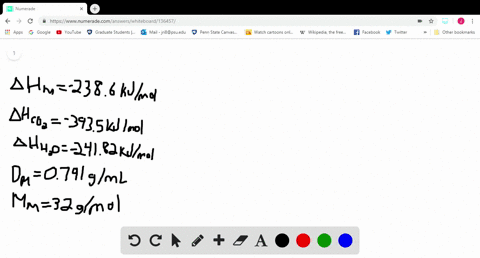Sign up for our free STEM online summer camps starting June 1st!View Summer Courses### Methanol (CH $_{3} \mathrm{OH}$ ) is used as a fu…

08:55Oklahoma State University

Need more help? Fill out this quick form to get professional live tutoring.

Get live tutoring
Problem 79

Ethanol $\left(\mathrm{C}_{2} \mathrm{H}_{5} \mathrm{OH}\right)$ is blended with gasoline as an automobile fuel. (a) Write a balanced equation for the combustion of liquid ethanol in air. (b) Calculate the standard enthalpy change for the reaction, assuming $\mathrm{H}_{2} \mathrm{O}(g)$ as a product.
(c) Calculate the heat produced per liter of ethanol by combustion of ethanol under constant pressure. Ethanol has a density of 0.789 $\mathrm{g} / \mathrm{mL}$ (d) Calculate the mass of $\mathrm{CO}_{2}$ produced per ky of heat emitted.

Check back soon!

## Discussion

You must be signed in to discuss.

## Video Transcript

No transcript available